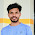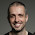Saturday, April 2, 2016

Python function arguments, *args and **kwargs

If you are new to Python, you have probably stumbled upon *args and **kwargs in someone else's code, on a forum or tutorial. This post explains what they are.

Types of function arguments in Python

Python functions accept 2 kinds of arguments: positional arguments and keyword arguments. With a few exceptions (explained below), when you define a function, you don't specify whether the arguments are positional or keyword. This is defined at the time the function is called, depending on the syntax used to pass the arguments.

Positional arguments

These arguments are identified based on the position they are supplied when calling the function.
``````def divide(dividend, divisor):
return dividend / divisor
``````

When called, the above function outputs:
``````divide(10,2)
5.0
``````

It knows 10 is the dividend and 2 is the divisor because of the order the arguments were provided.

Keyword arguments

Now let's suppose we want to call the above function inverting the argument order. We can do that using keyword arguments.
``````divide(divisor=2, dividend=10)
5.0
``````
Notice that the argument order was inverted but they were passed by name, so they were correctly identified.

In Python 3, by inserting "*" as a first argument, we force all following arguments to be called by name (as keyword arguments).
``````def divide(*, dividend, divisor):
return dividend / divisor
``````
The function above outputs:
``````divide(10,2)
TypeError: divide() takes 0 positional arguments but 2 were given

divide(dividend=10, divisor=2)
5.0
``````

What are *args and **kwargs?

*args and **kwargs allows you to pass an arbitrary number of arguments to a function without knowing them in advance.

The relevant syntax is the * and **. The names "args" and "kwargs" are only by convention. You could use *foo and **bar and have the same result. However, sticking to conventions makes it easier for us to read each other's code.

*args example

``````def print_args(*args):
for arg in args:
print(arg)
``````
Running the above function with an arbitrary number of arguments results in:
``````print_args('foo', 'bar', 5)
foo
bar
5
``````
There is no limit to the number of positional arguments you can provide.

**kwargs example

``````def print_kwargs(**kwargs):
for name, value in kwargs.items():
print('{0} = {1}'.format(name, value))
``````
If you are not familiar with the items() method, it's explained in this post.

Running the above function with an arbitrary number of arguments results in:
``````print_kwargs(meditation = 'zazen', minutes = 40)
meditation = zazen
minutes = 40
``````

Combining fixed arguments, *args and **kwargs

``````def print_args(fixed_arg, *args, **kwargs):
print(fixed_arg)
print(args)
print(kwargs)
``````
Running the above code outputs:
``````print_args('foo', 'bar', 'baz', meditation = 'zazen', minutes = 40)
foo
('bar', 'baz')
{'meditation': 'zazen', 'minutes': 40}
``````
Notice that:
• fixed_arg is a fixed positional argument
• args is a tuple of positional arguments
• kwargs is a dictionary of keyword arguments

1.1.